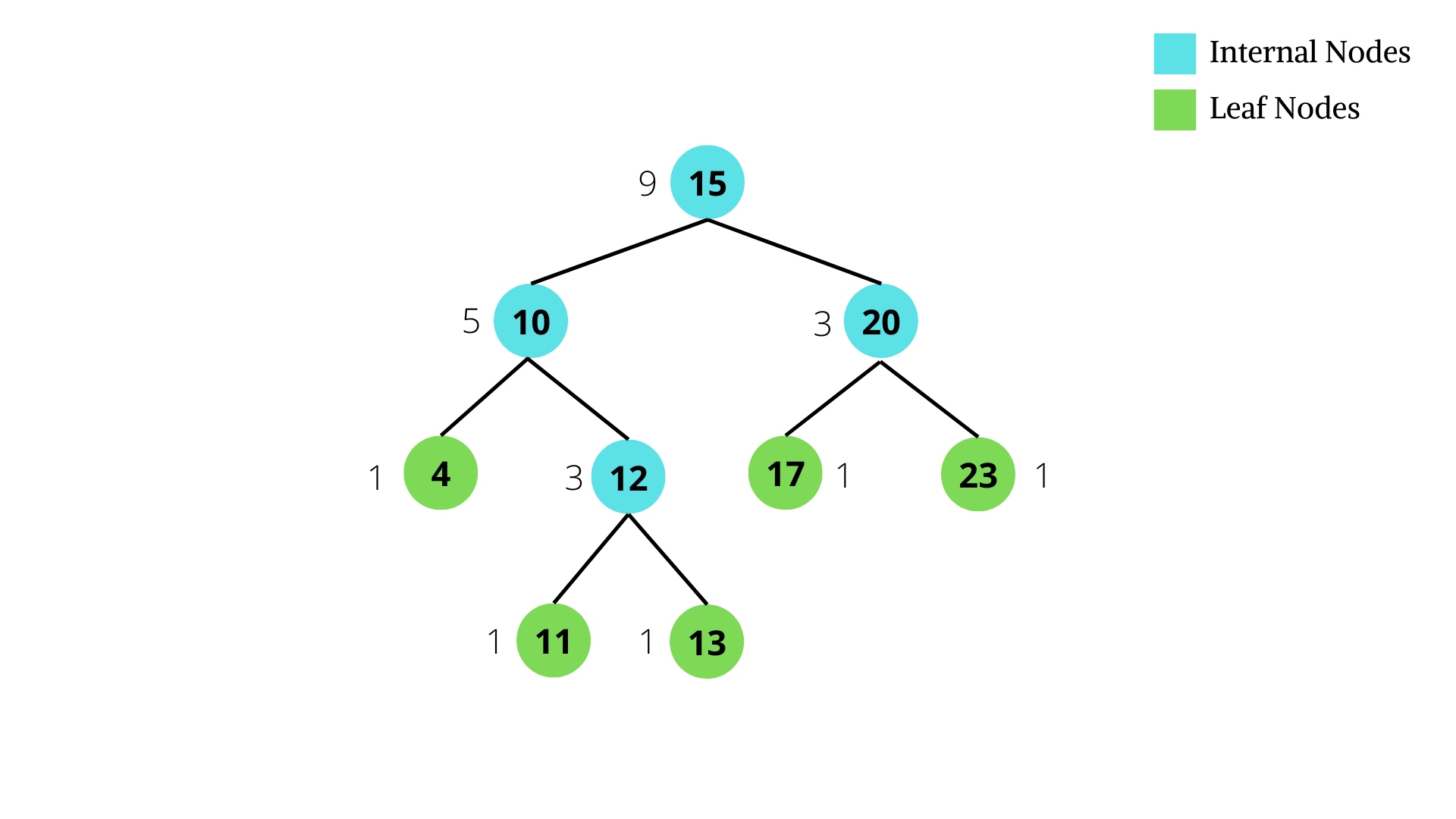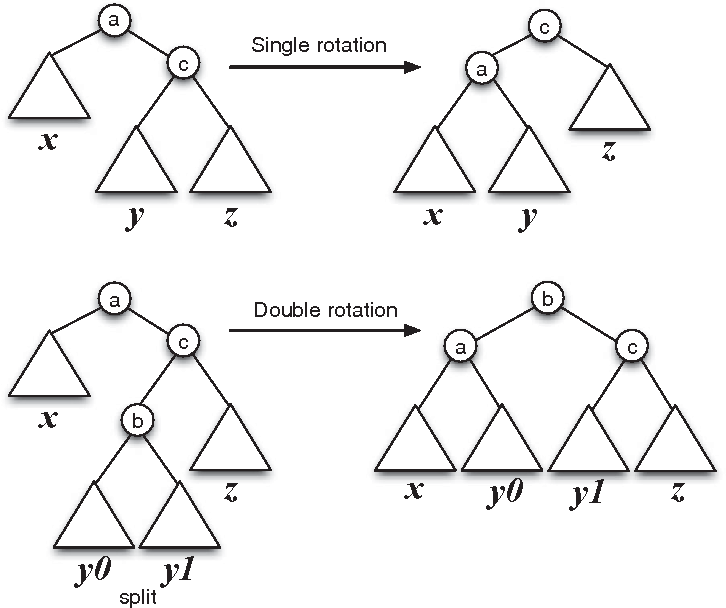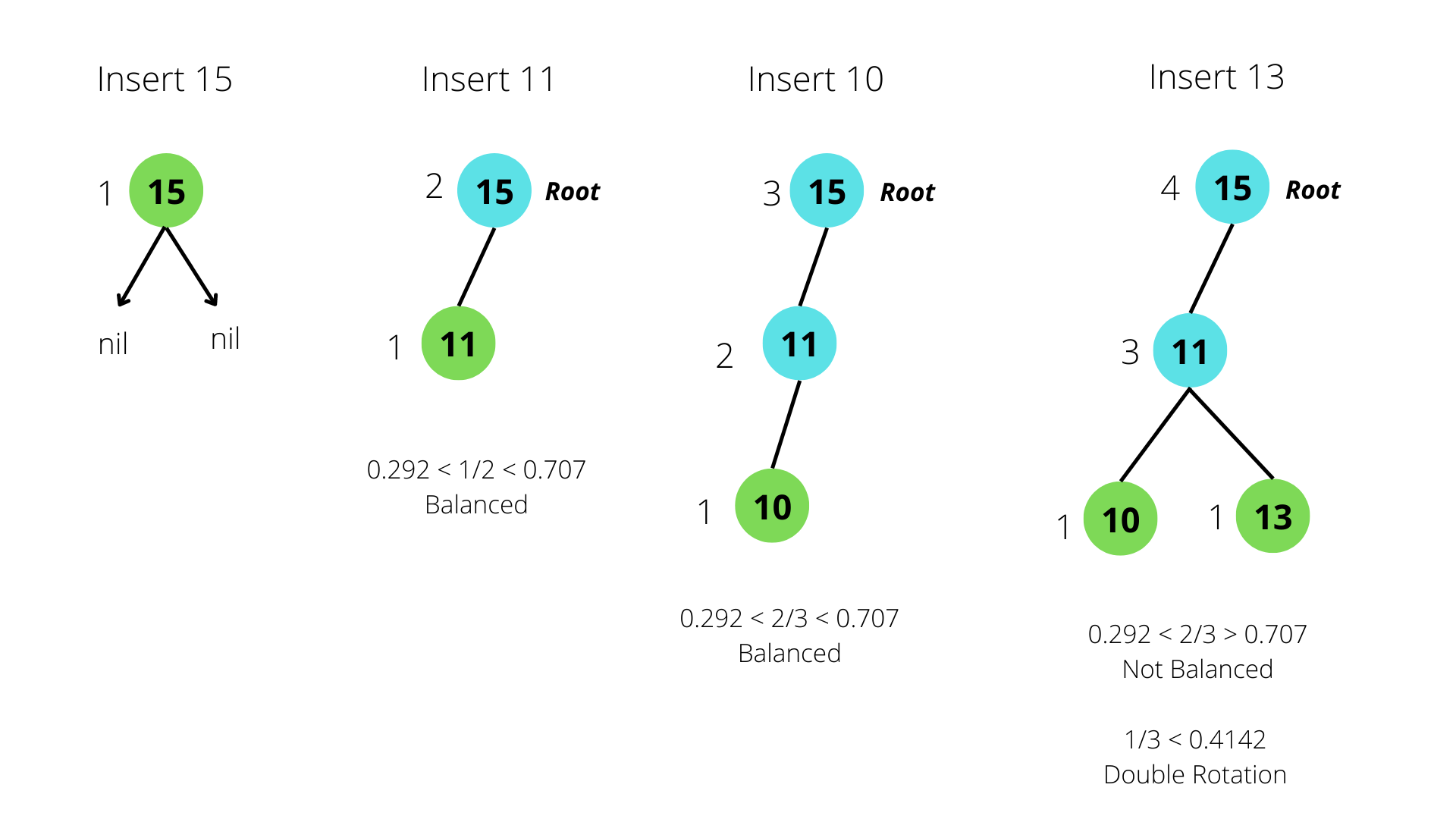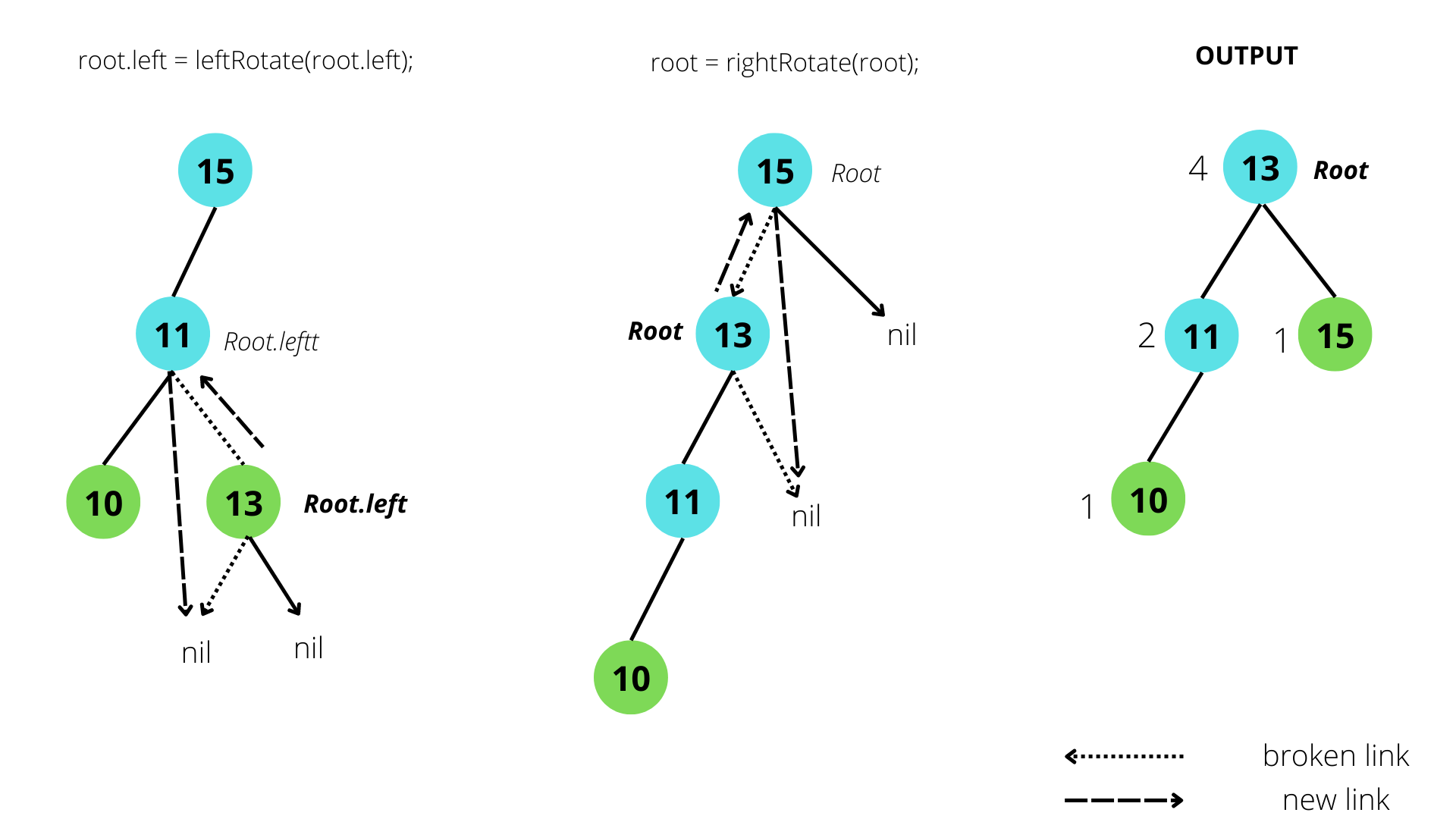×

Search anything:

# Weight Balanced Binary Tree

#### Algorithms Problems on Binary TreeGet this book -> Problems on Array: For Interviews and Competitive Programming

A self-balancing binary search tree is a type of binary tree that automatically adjusts its structure on insertion or deletion in order to maintain balance. Here, we will learn about Weight Balanced Binary Tree, which is a type of self-balancing binary search tree.

#### Table of Content:

1. Introduction
2. Features
3. Rebalance
4. Operations
a. Search
b. Rotation
c. Check Balance
d. Insertion
e. Deletion
5. Comparison

## Introduction

In a weight balanced binary tree, the aim is to balance the weight of a given tree in terms of number of leaves. The weight of a node depends on the weight of its children. A Binary Search Tree is weight balanced if for each node the number of nodes in the left subtree is at least half and at most twice the number of nodes in the right subtree.

A node in a weight-balanced binary search tree stores the sizes of subtrees in the nodes. A node will contain the following data:

• value of the element stored
• left and right pointer to children node or null
• weight of the node

The size of a leaf is usually considered as zero. The size of an internal node is the sum of sizes of its two children, plus one: (size[n] = size[n.left] + size[n.right] + 1). Based on the size, one defines the weight to be weight[n] = size[n] + 1.The above figure shows the weight of nodes along with its value. The node with the greatest weight is the root of the tree. The leaf nodes have size = 0 and weight = 1. The weight of the nodes decreases from root to leaf nodes.

Operations that modify the tree must make sure that the weight of the left and right subtrees of every node remain within some factor α of each other. Rebalancing of the tree is done through rotations and double rotations. Formally, node balance is defined as follows:

• A node is α-weight-balanced if α ≤ |Left(n)| / |Left(n)| + |Right(n)| ≤ 1 - α

Here, α is a numerical parameter to be determined when implementing weight balanced trees, where 2/11 < α < 1 - √2/2.

A node n is balanced if the number of leaf nodes in the left sub-tree and right sub-tree satisfy the following property:

leaves(n.left)/leaves(n.right) <= β ∈ (0, 1)

## Features:

1. Depth of a Weight-Balanced Binary Search Tree is always of the order log(N), where N is the total number of nodes inserted into the tree.

2. Search operations in a Weight Balanced Tree take O(Log(N)) time. The function is the same as the search operation in a Binary Search Tree. However, it is more efficient because the height of the tree is balanced.

3. Insertion operations take O(log(N)) time. It takes O(Log(N)) time to search for the appropriate location, O(1) time to insert, and O(Log(N)) time to rebalance.

4. Similar to the insertion operation, deletion operation takes O(Log(N)) time. Deletion requires O(Log(N)) time to search for the node, O(1) time to delete, and O(Log(N)) time to rebalance.

## Rebalance

After the tree is updated, it is checked to find out whether it remains α-balanced. Whenever the balance criterion at a node is violated, a single or double rotation, as depicted in the figure below, is performed to reestablish balance.Case 1:
weight(node->left) / weight (node) > α and weight(node->left) / weight(node) < 1 - α
In this case, the node is found to be balanced and no rotation is required.

Case 2:
weight(node->left) / weight (node) > α and weight(node->left) / weight(node) > 1 - α
In this case, the left node is too heavy because of an insertion into left subtree or a deletion from right subtree.
If the left subtree of the left child of the node is too heavy, a right rotation around the node is performed in such a case to reduce the weight of the left subtree. L(n) is made the root and the original root becomes the right child of L(n). The previous right child of L(n) (R(L(n))) becomes the left child of the original root.
Whereas, if the right subtree of the right child of the node is too heavy, a double rotation is performed. First a left rotation is performed on the left child of the root, followed by a right rotation on the root.

Case 3:
weight(node->left) / weight (node) < α and weight(node->left) / weight(node) < 1 - α
In this case, the right node is too heavy because of an insertion into right subtree or a deletion from left subtree.
A single rotation is performed if the right subtree of the right child of the node is too heavy. A left rotation around the node will certainly reduce the weight of the node’s right subtree, essentially removing R(n) and the subtree rooted in R(R(n)) from below n by making R(n) the root and the original root the left child of R(n).
In case the left child of the right subtree of the original root (L(R(n))) is too heavy, a double rotation is performed. A right rotation is performed on the right child of the root, followed by a left rotation on the root.

## Operations

### Defining the tree node

``````class Node {
Node left, right;
int weight, value;

/** Constructor **/
public Node(int val, int wt) {
this(val, wt, null, null);
}

/** Constructor **/
public Node(int val, int wt, Node left, Node right) {
this.value = val;
this.left = left;
this.right = right;
this.weight = wt;
}
}
``````

### 1. Search

The algorithm takes the root of the tree and value to be searched as parameters.

##### Steps:
1. Construct a Weight-Balanced Binary Search Tree.
2. Insert root of tree and value to be searched as parameters in the search function and call it.
3. If root is null, return null.
4. If the root node contains the value, return the node.
5. If the value is greater than node value, call the search function with the right child as root. The right subtree contains elements greater than the root node.
6. Similarly, if the value is less than the node value, call the search function with the left child as root since the left subtree contains elements smaller than the root node.
##### Pseudocode:

FUNCTION search(node, value)
IF node is null
RETURN null
IF value of node is equal to the required value
RETURN node
IF value of node is greater than required value
RETURN search(right child, value)
IF value of node is smaller than required value
RETURN search(left child, value)

##### Implementation:
``````    public Node search(Node root, int value)
{
// Base Cases: root is null or value is present at root
if (root==null)
return null;

if(root.value==value)
return root;

// value is greater than root's val
if (root.value < value)
return search(root.right, value);

// value is smaller than root's value
return search(root.left, value);
}
``````

### 2. Rotation

In order to rebalance an imbalanced tree, left or right rotation is performed on the node. The imbalanced node is taken as a parameter.

##### Steps:

Left Rotation:

1. Store the root node in temp variable.
2. Make the right child of root node the root.
3. Make the left child of the current root the right child of temp (previous root).
4. Make temp the left child of current root.
5. Update the respective weights.

Right Rotation:

1. Store the root node in temp variable.
2. Make the left child of root node the root.
3. Make the right child of the current root the left child of temp (previous root).
4. Make temp the right child of current root.
5. Update the respective weights.
##### Pseudocode:

LEFT ROTATION:
SET temp as root node
SET root as root->right
SET temp->right as root->left
SET left->left as temp
UPDATE weights of root and temp
RETURN root

RIGHT ROTATION:
SET temp as root node
SET root as root->left
SET temp->left as root->right
SET left->right as temp
UPDATE weights of root and temp
RETURN root

##### Implementation:
``````    public Node leftRotate(Node root) {
Node temp = root;
root = root.right;
temp.right = root.left;
root.left = temp;
root.weight = temp.weight;
temp.weight = temp.left.weight + temp.right.weight + 1;
return root;
}

public Node rightRotate(Node root) {
Node temp = root;
root = root.left;
temp.left = root.right;
root.right = temp;
root.weight = temp.weight;
temp.weight = temp.left.weight + temp.right.weight + 1;
return root;
}
``````

### 3. Check Balance

To check if a particular node is balanced of not. If it is not balanced, the node is rotated. The function takes a node as parameter.

##### Steps:
1. Find the ratio of weight of left subtree of root to weight of root.
2. If ratio is more than alpha value (0.293), the left subtree is too heavy.
3. If the ratio of weight of left subtree of left child of root to the weight of left child of root is greater than 0.414, perform single right rotation. Else perform double rotation - left followed by right.
4. If ratio is less than 1 - alpha value (0.707), the right subtree is too heavy.
5. If the ratio of weight of left subtree of right child of root to the weight of right child of root is less than 0.585, perform single left rotation. Else perform double rotation - right followed by left.
6. Return the balanced node.
##### Pseudocode:

SET wbal as weight(root -> left)/weight(root)
IF wbal > 0.707
IF weight(root->left->left)/weight(root->left) > 0.414
RETURN rightRotate(root)
ELSE
UPDATE root->left = leftRotate(root->left)
RETURN rightRotate(root)
ELSE IF wbal < 0.292
IF weight(root->right->left)/weight(root->right) < 0.585
RETURN leftRotate(root)
ELSE
UPDATE root->right = rightRotate(root->right)
RETURN leftRotate(root)

##### Implementation:
``````    public Node checkBalance(Node root) {
float wbal = (float) root.left.weight / (float) root.weight;
if (wbal > 0.70711 && root.left != nil) {

if ((float) root.left.left.weight / (float) root.left.weight > 0.414213)
root = rightRotate(root);
else {
root.left = leftRotate(root.left);
root = rightRotate(root);
}
} else if (wbal < 0.29289 && root.right != nil) {
if ((float) (root.right.left.weight / (float) root.right.weight) < 0.585786)
root = leftRotate(root);
else {
root.right = rightRotate(root.right);
root = leftRotate(root);
}
}
return root;
}
``````

### 4. Insertion

The algorithm takes a value, weight, and root node as parameters.

##### Steps:
1. If the root is null, insert a new node with given value and weight.
2. If the value of the root node is greater than the given value, call the insert function with the left child as root. The new node will be added to the left subtree of root.
3. If the value of the root node is lesser than the given value, call the insert function with the right child as root. The new node will be added to the right subtree of root.
4. Return the balanced root node.
##### Pseudocode:

FUNCTION insert(value, weight, root)
IF root is null
RETURN new leaf node with value and weight
IF value of root is lesser than given value
UPDATE left subtree of root = insert(value, weight, current left child)
IF value of root is greater than given value
UPDATE right subtree of root = insert(value, weight, current right child)
UPDATE weight of root
CHECK balance of root and update root to balanced root
RETURN balanced root

##### Implementation:
``````   public Node insert(int X, Node T) {
if (T == nil)
return new Node(X, 1, nil, nil);
if (X < T.element) {
T.left = insert(X, T.left);
} else if (X > T.element) {
T.right = insert(X, T.right);
}
T.weight = T.left.weight + T.right.weight + 1;
return checkBalance(T);
}
``````

### 5. Deletion:

To delete a node from the tree, the function takes the key value and root node as parameter.

##### Steps:
1. If the root is null, print Key not found and return root.
2. If the value of the root node is greater than the key value, call the delete function with the left child as root.
3. If the value of the root node is less than the key value, call the delete function with the right child as root.
4. If left child of the root is nil, make the right child of root as root.
5. If right child of the root is nil, make the left child of root as root.
6. If left child weighs more than right child, perform right rotation. Call delete function on the right child of root. Else perform left rotation and call delete function on the left child of root.
7. Update weight if root is not nil. Check Balance.
8. Return balanced root.
##### Pseudocode:

IF root is nil
RETURN root
IF key < root->element
UPDATE root->left = delete(key, root->left)
ELSE IF key > root->element
UPDATE root->right = delete(key, root->right)
ELSE IF root has no left child
UPDATE root = root->right
ELSE IF root has no right child
UPDATE root = root->right
ELSE IF weight(root->left) > weight(root->right)
PERFORM rightRotate(root)
UPDATE root->right = delete(key, root.right)
ELSE
PERFORM leftRotate(root)
UPDATE root->left = delete(key, root.left)

IF root is not nil
UPDATE weight(root)
CHECK balance and update root

RETURN balanced root

##### Implementation:
``````    public Node delete(int key, Node root) {
if (root == nil) {
return root;
}
if (key < root.element)
root.left = delete(key, root.left);
else if (key > root.element)
root.right = delete(key, root.right);

else if (root.left == nil)
root = root.right;  // root contains key and has one child - right
else if (root.right == nil)
root = root.left;   // root contains key and has one child - left

else if (root.left.weight > root.right.weight) {
root = rightRotate(root);
root.right = delete(key, root.right);
} else {
root = leftRotate(root);
root.left = delete(key, root.left);
}

if (root != nil) {
root.weight = root.left.weight + root.right.weight + 1;
root = checkRotation(root);
}

return root;
}
``````

### Example:

Input: [15, 11, 10, 13]

Inputs are added one at a time. The tree remains balanced for inputs 15, 11 and 10. However, on insertion of 13, the left subtree becomes too heave. Double rotation is performed to restore balance.## Comparison

Height-Balanced Tree Weight-Balanced Tree
A binary tree is height-balanced if there is no considerable difference between the height of the left and right subtrees. A binary tree is weight balanced if the weight of the right and left subtree in each node differ by at most one.
Height of a height-balanced tree with N nodes is O(logN) Height of a weight-balanced tree with N nodes is O(logN)
The restructuring operation on a node with n descendants happens every 2-O(lg n) operation. The restructuring operation on a node with n descendants happens every O(1/n) operation.
Insertion and Deletion both take O(LogN) time Insertion and Deletion both take O(LogN) time
Examples: AVL Tree, Red-Black Tree, Splay Tree Examples: BB(α) Tree#### Astha Jain

Astha Jain is a BE student of Information Technology (IT) at Savitribai Phule Pune University.